﻿ 基于可视化技术设计实现雷达数据处理软件平台

# 基于可视化技术设计实现雷达数据处理软件平台Based on the Visualization Technology, Design and Implement a Radar Data Processing Software Platform

Abstract: In order to improve the efficiency of radar data processing, a software platform for radar data processing is designed and implemented based on visualization technology. The design of two core components of the platform, namely, the waveform chart and the text window, is emphasized. The waveform chart is used for the observation of data as a whole as well as in detail. The operations imposed on the waveform curve include panning freely, scaling horizontally or vertically, viewing data point, etc. And the waveform chart data can be dynamically selected based on a proposed data selection algorithm. An alternate visualization method of viewing and processing data lies in the design of the text window where a shortcut menu is utilized to enhance the interactivity of the human-computer operation. A fast and efficient drawing algorithm is adopted to improve the drawing speeds of both the waveform chart and the text window. The application results of data processing for a certain type of monopulse radar show that using this software platform improves the data processing efficiency.

1. 引言

2. 可视化雷达数据处理软件平台设计Figure 1. The software platform architecture for visual radar data processing

2.1. 设计实现波形图

2.1.1. 注册窗口类

ATOM RegisterClassEx(CONST WNDCLASSEX *lpwcx)；

WNDCLASSEX结构体中包含了窗口类的信息，其定义如下：

typedef struct_WNDCLASSEX {

UINT cbSize;

UINT style;

WNDPROC lpfnWndProc;

int cbClsExtra;

int cbWndExtra;

HINSTANCEhInstance;

HICON hIcon;

HCURSOR hCursor;

HBRUSH hbrBackground;

LPCTSTR lpszClassName;

HICON hIconSm;

} WNDCLASSEX, *PWNDCLASSEX;

cbSize：给出本结构体的以字节为单位的大小。

style：指定窗口类的风格。

lpfnWndProc：指向窗口过程的指针。

cbClsExtra、cbWndExtra：分别指定本窗口类后面、实例后面额外分配的字节数，系统会将这些字节的内容全部初始化为零。

hInstance：本类窗口实例的句柄。

hIcon：窗口类图标的句柄。

hCursor：窗口类光标的句柄。

hbrBackground：窗口类背景画刷的句柄。

lpszClassName：指向一个以零字符结尾的字符串，该字符串代表了窗口类的类名。

hIconSm：代表一个与窗口类关联的小图标的句柄。

2.1.2. 创建窗口

HWND CreateWindowEx(DWORD dwExStyle, LPCTSTR lpClassName,

LPCTSTR lpWindowName, DWORD dwStyle, int x, int y, int nWidth, int nHeight,

CreateWindowEx函数的参数较多，各个参数的含义简述如下。

dwExStyle：指定所创建窗口的扩展样式，具体样式参见文献  。

lpClassName、lpWindowName：二者均为指向以零字符结尾的字符串，两个字符串分别标识窗口类的类名和窗口的标题。

dwStyle：指定所创建窗口的风格。

x、y：指定窗口的最初位置。对于层叠式或弹出式窗口来说，它们分别表示屏幕坐标系中窗口左上角的x、y坐标；而对于子窗口来说，它们则分别表示窗口左上角相对于其父窗口客户区的x、y坐标。

nWidth、nHeight：以设备单位表示的窗口的宽度和高度。

hWndParent：指定父窗口的句柄。

hInstance：窗口关联模块的实例句柄。

lpParam：窗口创建后，窗口过程将接收到WM_CREATE消息，该消息的LPARAM类型的参数是一个指向CREATESTRUCT类型结构体的指针，该结构体的LPVOID成员即等于此lpParam。若创建的是MDI的客户窗口，则lpParam为一指向CLIENTCREATESTRUCT结构体的指针。

2.1.3. 实现窗口消息分发机制

#include

#include

using namespace std;

class CPlot

{

public:

CPlot(HWND hwnd);

virtual ~CPlot();

protected:

HWNDm_hWnd;

static vector >m_svpPlots;

static LRESULT CALLBACK WndProc(HWND hwnd, UINT message, WPARAM wParam, LPARAM lParam);

LRESULT_WndProc(UINT message, WPARAM wParam, LPARAM lParam);

……

};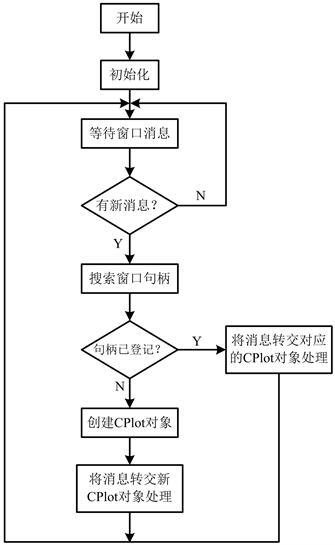Figure 2. The message distribution mechanism of the waveform chart window

2.2. 设计实现文本窗

1) 用户在文本窗的字段区即标题区按下鼠标右键；

2) 程序代码判断鼠标指针指向了哪种数据(用指针指向的数据列来区分)；

3) 弹出相应的快捷菜单，例如图3表示用户在R数据列上按下了鼠标右键；

4) 执行相应的菜单命令，完成诸如角度弧度互化、查看数据波形、查看数据差分波形等功能。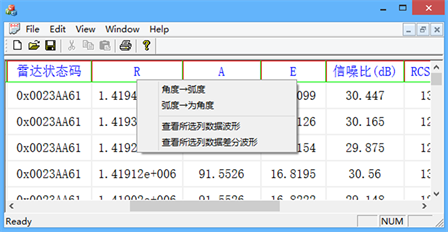Figure 3. An example of the text window design

3. 关键技术

3.1. 波形图快速高效绘制算法

3.1.1. 算法推导

$\left({x}_{0},{y}_{0}\right),\left({x}_{1},{y}_{1}\right),\cdots ,\left({x}_{I},{y}_{I}\right),\cdots ,\left({x}_{N-1},{y}_{N-1}\right)$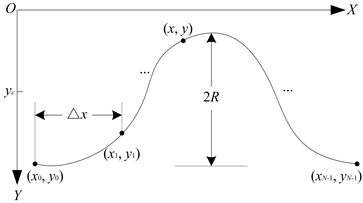Figure 4. A drawing schematic in the application client area

$\left\{\begin{array}{l}I=\frac{x-{x}_{0}}{\Delta x}\\ Y={Y}_{c}-\frac{y-{y}_{c}}{2R}ly\end{array}\text{ }\left(\Delta x>0,\text{\hspace{0.17em}}ly>0,\text{\hspace{0.17em}}R>0\right)$

$\begin{array}{l}{Y}_{c}=\left({Y}_{\mathrm{max}}+{Y}_{\mathrm{min}}\right)/2\\ ly={Y}_{\mathrm{max}}-{Y}_{\mathrm{min}}\end{array}$

3.1.2. 自由平移、水平缩放、竖直缩放关键技术

3.1.3. 水平方向缩放图形

$\left\{\begin{array}{l}\frac{x-{x}_{0}}{\Delta x}=\frac{{x}^{\prime }-{{x}^{\prime }}_{0}}{\Delta {x}^{\prime }}\\ {x}^{\prime }=x,\text{ }{y}^{\prime }=y\end{array}$

3.1.4. 竖直方向缩放图形

$\left\{\begin{array}{l}\frac{y-{y}_{c}}{R}=\frac{{y}^{\prime }-{{y}^{\prime }}_{c}}{{R}^{\prime }}\\ {x}^{\prime }=x,\text{ }{y}^{\prime }=y\end{array}$

3.1.5. 自由缩放图形

$\left\{\begin{array}{l}\frac{l-{x}_{0}}{\Delta x}=\frac{{x}_{1}-{{x}^{\prime }}_{0}}{\Delta {x}^{\prime }}\\ \frac{r-{x}_{0}}{\Delta x}=\frac{{x}_{2}-{{x}^{\prime }}_{0}}{\Delta {x}^{\prime }}\end{array}\text{ }\text{ }\text{ }\left\{\begin{array}{l}\frac{t-{y}_{c}}{R}=\frac{{y}_{1}-{{y}^{\prime }}_{c}}{{R}^{\prime }}\\ \frac{b-{y}_{c}}{R}=\frac{{y}_{2}-{{y}^{\prime }}_{c}}{{R}^{\prime }}\end{array}$

3.1.6. 查看图形上某点数据

$\left\{\begin{array}{c}I=\frac{x-{x}_{0}}{\Delta x}\\ Y={Y}_{c}-\frac{y-{y}_{c}}{2R}ly\end{array}\text{ }\left(\Delta x>0,ly>0,R>0\right)$

3.1.7. 确定无效矩形包含的数据索引Figure 5. Determines the data range and axis scale range associated with the invalid rectangle

$\text{iIndexBeg}=\mathrm{min}\left(\mathrm{max}\left(0,\mathrm{int}\left(\frac{{s}_{1}-{x}_{0}}{\Delta x}\right)\right),N-1\right);$

$\text{iTemp}=\mathrm{int}\left(\frac{{s}_{2}-{x}_{0}}{\Delta x}\right);$

$\text{if}\left({s}_{2}>{x}_{0}且{s}_{2}不是整数\right)$

$++\text{iTemp};$

$\text{iIndexEnd}=\mathrm{max}\left(\mathrm{min}\left(N-1,\text{iTemp}\right),0\right);$

$\text{if}\left(\text{iIndexBeg}<\text{iIndexEnd}\right)$

$\left\{//绘制该部分图形\right\}$

3.1.8. 确定无效矩形包含的OX轴刻度

${k}_{\text{1}}=\mathrm{int}\left(\frac{{s}_{1}-{x}_{0}}{\Delta {p}_{x}}\right);{k}_{\text{2}}=\mathrm{int}\left(\frac{{s}_{2}-{x}_{0}}{\Delta {p}_{x}}\right);$

$\text{if}\left({s}_{1}>{x}_{0}且{s}_{1}不是整数\right)$

$++{k}_{\text{1}};$

$\text{if}\left({s}_{2}<{x}_{0}且{s}_{2}不是整数\right)$

$--{k}_{\text{2}};$

$\text{if}\left({k}_{1}\le {k}_{2}\right)$

$\left\{//绘制OX轴刻度线\right\}$

3.1.9. 确定无效矩形包含的OY轴刻度

${m}_{\text{1}}=\mathrm{int}\left(\frac{{s}_{1}-{y}_{c}}{\Delta {p}_{y}}\right);{m}_{\text{2}}=\mathrm{int}\left(\frac{{s}_{2}-{y}_{c}}{\Delta {p}_{y}}\right);$

$\text{if}\left({s}_{1}>{y}_{c}且{s}_{1}不是整数\right)$

$++{m}_{1};$

$\text{if}\left({s}_{2}<{y}_{c}且{s}_{2}不是整数\right)$

$--{m}_{2};$

$\text{if}\left({m}_{1}\le {m}_{2}\right)$

$\left\{//绘制OY轴刻度线\right\}$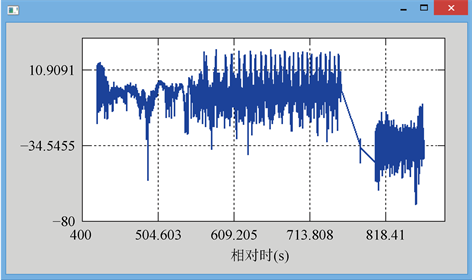Figure 6. An example of the application of the fast and efficient drawing algorithm for a waveform chart

3.1.10. 快捷菜单设计的关键技术

LRESULT CALLBACK WindowProc(

HWND hwnd, // 窗口句柄

WPARAM wParam, // 窗口句柄

LPARAM lParam // 鼠标指针位置

);

xPos = GET_X_LPARAM(lParam); // 获取鼠标位置的x坐标

yPos = GET_Y_LPARAM(lParam); // 获取鼠标位置的x坐标

xPos, yPos, 0, hwnd, NULL);

3.2. 图形选择算法Figure 7. A schematic of the graph selection algorithm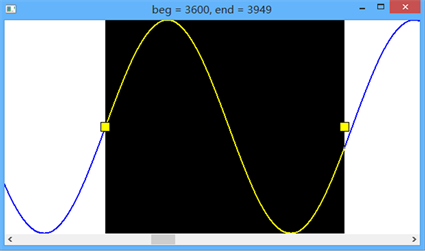Figure 8. A graphic selection example

4. 性能评估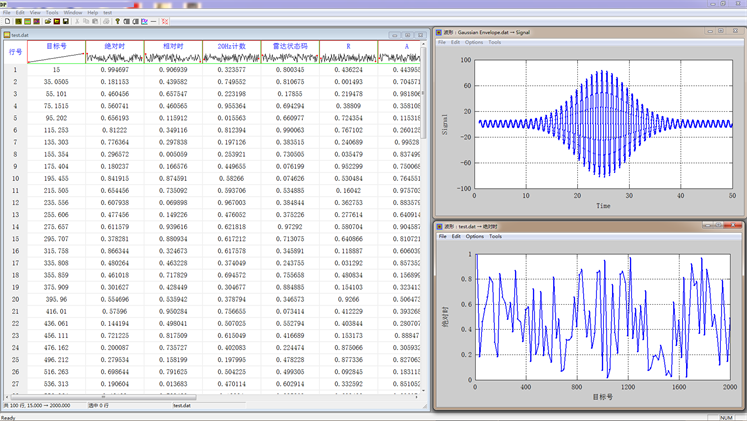Figure 9. The visual interface of the data processing platform

5. 结束语

 Skolnik, M.I. (2007) Introduction to Radar Systems. 3rd Edition, Publishing House of Electronics Industry, Beijing, 772.

 张威. MATLAB外部接口编程[M]. 第1版. 西安: 西安电子科技大学出版社, 2004: 208.

 霍亚飞. Qt Creator快速入门[M]. 第3版. 北京: 北京航空航天大学出版社, 2017: 515.

 周明扬. Visual C++界面编程技术[M]. 第1版. 北京: 北京希望电子出版社, 2003.

 Horton, I. Visual C++ 2012入门经典[M]. 第6版. 北京: 清华大学出版社, 2013.

 Lippman, S.B., Lajoie, J. and Moo, B.E. (2007) C++ Primer. 4th Edition. Post & Tel-ecom Press Co., LTD., Beijing, 885.

 Richter, J., Nasarre, C. Windows核心编程[M]. 第1版. 北京: 清华大学出版社, 2008: 770.

 Microsoft Corporation. The October 2001 Release of the MSDN Library [DB/CD]. 2001.

 李婷, 张志民, 李红梅. 火箭飞行任务动态故障树分析与管理系统设计与实现[J]. 东风航天, 2017, 29(5): 52-55.

 李婷, 张志民, 贾森林. 基于“计算机+人”联合判读的火箭故障智能诊断系统设计[J]. 导弹试验技术, 2016(2): 1-7.

 张志民, 欧建平, 皇甫堪. 一种Windows下实时高效图形绘制算法[J]. 现代电子技术, 2010(16): 43-46.

 程佩青. 数字信号处理教程MATLAB版[M]. 第5版. 北京: 清华大学出版社, 2017: 675.

 Proakis, J. G., Manolakis, D. G. 数字信号处理: 原理、算法与应用[M]. 第3版. 张晓林, 译. 北京: 电子工业出版社, 2004: 827.

Top